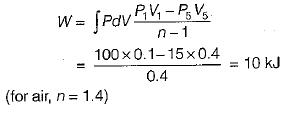Courses

# Work And Heat Transfer - 1

## 10 Questions MCQ Test Topicwise Question Bank for GATE Mechanical Engineering | Work And Heat Transfer - 1

Description
This mock test of Work And Heat Transfer - 1 for Mechanical Engineering helps you for every Mechanical Engineering entrance exam. This contains 10 Multiple Choice Questions for Mechanical Engineering Work And Heat Transfer - 1 (mcq) to study with solutions a complete question bank. The solved questions answers in this Work And Heat Transfer - 1 quiz give you a good mix of easy questions and tough questions. Mechanical Engineering students definitely take this Work And Heat Transfer - 1 exercise for a better result in the exam. You can find other Work And Heat Transfer - 1 extra questions, long questions & short questions for Mechanical Engineering on EduRev as well by searching above.
QUESTION: 1

### A closed system receives 60 kJ heat but its internal energy decreases by 30 kJ, Then the work done by the system is

Solution: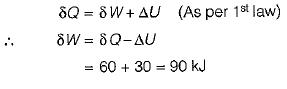QUESTION: 2

### If the work done on a closed system is 20 kJ/kg, and 40 kJ/kg heat is rejected from the system, its internal energy decreases by

Solution:

As per first law of thermodynamics: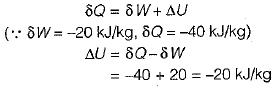QUESTION: 3

### The integrating factor of quasi-static displacement work is

Solution:

Quasistatic work,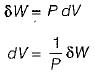An inexact different dW when multiplied by an integrating factor 1/P becomes an exact differential dV.
(Point function) = (Integrating factor) x (Path function)

QUESTION: 4

The integrating factor of reversible heat transfer is

Solution: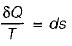QUESTION: 5

Heat transferred to a closed stationary system at constant volume is equal to

Solution: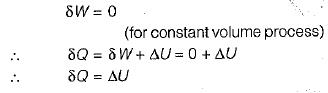QUESTION: 6

Which among the following is not a boundary phenomenon?

Solution:
QUESTION: 7

In a general compression process, 2 kJ of mechanical work is supplied to 4 kg of fluid and 800 J of heat is rejected to the cooling jacket. The change in specific internal energy would be

Solution: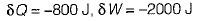QUESTION: 8

Which among the following is not true?

Solution:
QUESTION: 9

The following data refer to a 6-cylinder, single- acting, two-stroke diesel engine:
Speed-250 rpm
Cylinder diameter - 0.7 m
Stroke of a piston - 1.2 m
Area of indicator diagram - 5.5 x 10-4 m2
Length of diagram - 0.06 m
Spring value -144 MPa per m

What is the net rate of work transfer from the gas to the pistons in kW?

Solution: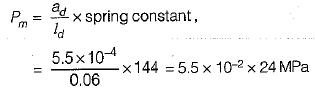Net work transfer,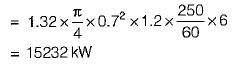QUESTION: 10

A piston-cylinder device with air at an initial temperature of 289C undergoes an expansion process for which pressure and volume are related as given below: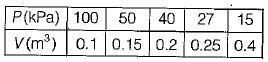What is the work done by the system?

Solution: